• 预科 学习编程之前对于编程逻辑理解 全名叫什么：使用java理解程序逻辑 一些PPT 老师上课用的
• 逻辑斯蒂回归是一个非常经典的二项分类模型，也可以扩展为多项分类模型。其在应用于分类时的过程一般如下，对于给定的数据集，首先根据训练样本点学习到参数w,b;再对预测点分别计算两类的条件概率，将预测点判为概率...
逻辑斯蒂回归是一个非常经典的二项分类模型，也可以扩展为多项分类模型。其在应用于分类时的过程一般如下，对于给定的数据集，首先根据训练样本点学习到参数w,b;再对预测点分别计算两类的条件概率，将预测点判为概率值较大的一类。
1、线性模型  逻辑斯蒂回归属于对数线性模型，那什么是对数线性模型？首先我们介绍下线性模型。  给定包含d个属性的变量x=(

x1,x2,...,xd
$x_1,x_2,...,x_d$),

xi
$x_i$表示在第i个属性上的取值，线性模型通过学得一个对属性分量的线性组合来进行预测的函数，即：

f(x)=w1x1+w2x2+⋯+wdxd+b
$f(x)=w_1x_1+w_2x_2+\cdots+w_dx_d+b$

写成向量形式为：

f(x)=wTx+b
$f(x)=w^Tx+b$

线性模型形式简单，易于建模，很多不错的非线性模型都是以线性模型为基础，通过层次组合或高维映射形成。此外，向量w作为各分量的权值，可以很直观地解释各属性在模型分类中的重要性，例如：

f好瓜=0.2⋅x色泽+0.5⋅x根蒂+0.3⋅x敲声
$f_{好瓜}=0.2\cdot x_{色泽}+0.5\cdot x_{根蒂}+0.3\cdot x_{敲声}$ +1

显然，根蒂对判断是否为好瓜的影响最大。  当我们给定样例点

(x,y)
$(\mathbf {x,y})$， 若线性模型对给定样本点的预测值

f(x)
$f(x)$逼近真实值y时，就形成了线性回归模型，记为：

y=wTx+b
$y=w^Tx+b$

线性回归模型表征了输入x与输出y的一种线性关系，我们还可以定义输入x与输出y的函数g(y)的一种线性关系，如：

lny=wTx+b
$lny=w^Tx+b$

就是一种对数线性回归，使x与输出的对数形成线性关系，实际上使用w^Tx+b的指数

ewTx+b
$e^{w^Tx+b}$来逼近输出。  考虑一般性，我们记：

g(y)=wTx+b
$g(y)=w^Tx+b$

其中，g(y)应满足单调可微的性质。我们将这样的模型称为“广义线性模型”，对数线性模型即g函数取对数函数的情况。  2、逻辑斯蒂回归模型  开始提到了逻辑斯蒂回归是一种对数线性模型，也就是说其输入与输出的对数函数成线性关系，实际上，它们满足如下关系：

logP(Y=1|x)P(Y=0|x)=wTx+b
$log \frac {P(Y=1|x)}{P(Y=0|x)}=w^Tx+b$

关系如何得来的?  根据上面提到的广义线性模型，对预测值的对数函数，需要满足单调可微的性质，且方便进行二项分类，于是选取了S形曲线Sigmoid函数作为

g−(⋅)
$g^-(\cdot)$函数，如下：

y=11+e−z
$y=\frac{1}{1+e^{-z}}$

图形如下：我们将输入的线性组合代替Sigmoid函数中的输入，得到逻辑斯蒂回归模型。  逻辑斯蒂回归模型是如下的条件概率分布：

P(Y=1|x)=11+e−(w⋅x+b)=e(w⋅x+b)1+e(w⋅x+b)
$P(Y=1|x)=\frac{1}{1+e^-{(w\cdot x+b)}}=\frac {e^{(w\cdot x+b)}}{1+e^{(w\cdot x+b)}}$

P(Y=0|x)=1−P(Y=1|X)=11+e(w⋅x+b)
$P(Y=0|x)=1-P(Y=1|X)=\frac {1}{1+e^{(w\cdot x+b)}}$

显然，条件概率分布与曲线是一致的，即当输入越小时，取正例的概率趋近于0，取反例的概率趋近于1；当输入越大时，取正例的概率则趋近于1，取反例的概率趋近于0.  记：

y=P(Y=1|x)
$y=P(Y=1|x)$

1−y=P(Y=0|x)
$1-y=P(Y=0|x)$

则有：

y1−y=P(Y=1|x)P(Y=0|x)=ew⋅x+b
$\frac y{1-y}=\frac{P(Y=1|x)}{P(Y=0|x)}=e^{{w\cdot x+b}}$

即：

logy1−y=w⋅x+b
$log \frac {y}{1-y}={w\cdot x+b}$

从而得出关系。这里我们把

logy1−y=w⋅x+b
$log \frac {y}{1-y}={w\cdot x+b}$ 称作对数几率，表示一个事件预测为正例与反例的比值的对数。
3、模型参数估计  我们得到逻辑斯蒂回归模型的表示，也即条件概率分布后，需要得到参数w,b的值才能对未知输入点进行预测。  对于逻辑斯蒂回归模型，一般使用极大似然估计的方法估计模型参数。转化为对数似然函数后，问题就变成了带有参数的求似然函数值最大值的最有问题。因为这是属于无约束优化问题，一般采用梯度下降法、牛顿法、拟牛顿法等方法来进行求解，得到参数估计值

w^,b^
$\hat w,\hat b$ 后，代入条件概率分布公式：

P(Y=1|X)=e(w^⋅x+b^)1+e(w^⋅x+b^)
$P(Y=1|X)=\frac {e^{(\hat w\cdot x+\hat b)}}{1+e^{(\hat w\cdot x+\hat b)}}$

P(Y=0|X)=11+e(w^⋅x+b^)
$P(Y=0|X)=\frac {1}{1+e^{(\hat w\cdot x+\hat b)}}$

即可实现对二项分类的预测。
4、多项逻辑斯蒂回归  二项逻辑斯蒂回归也可以推广到多项逻辑斯蒂回归，从而应用到多类分类问题中。  假设类别集合为{1,2,…,K}，多项逻辑斯蒂回归模型可以写作：

P(Y=k)=exp(wk⋅x)1+∑K−1k=1exp(wk⋅x)
$P(Y=k)=\frac {exp(w_k\cdot x)}{1+\sum_{k=1}^{K-1}exp(w_k\cdot x)}$

P(Y=K|x)=11+∑K−1k=1exp(wk⋅x)
$P(Y=K|x)=\frac {1}{1+\sum_{k=1}^{K-1}exp(w_k\cdot x)}$

可以满足

∑Kk=1P(Y=k|x)=1
$\sum_{k=1}^KP(Y=k|x)=1$

展开全文线性模型
• 基础的java语言，适合初学者更好的理解java的逻辑思想，含有简单的程序编写便于更好的理解，欢迎您的下载。。。
• 下面小编就为大家带来一篇深入理解逻辑表达式的用法 与或非的用法。小编觉得挺不错的，现在就分享给大家，也给大家做个参考。一起跟随小编过来看看吧
• ## 逻辑回归的理解

万次阅读 多人点赞 2018-03-15 21:01:38
逻辑回归(Logistic Regression) 1. 回归(Regression) - 回归，我的理解来说，其直观的理解就是拟合的意思。我们以线性回归为例子，在二维平面上有一系列红色的点，我们想用一条直线来尽量拟合这些红色的点，这...
逻辑回归(Logistic Regression)

1. 回归(Regression)

回归，我的理解来说，其直观的理解就是拟合的意思。我们以线性回归为例子，在二维平面上有一系列红色的点，我们想用一条直线来尽量拟合这些红色的点，这就是线性回归。回归的本质就是我们的预测结果尽量贴近实际观测的结果，或者说我们的求得一些参数，经过计算之后的预测结果尽可能接近真实值。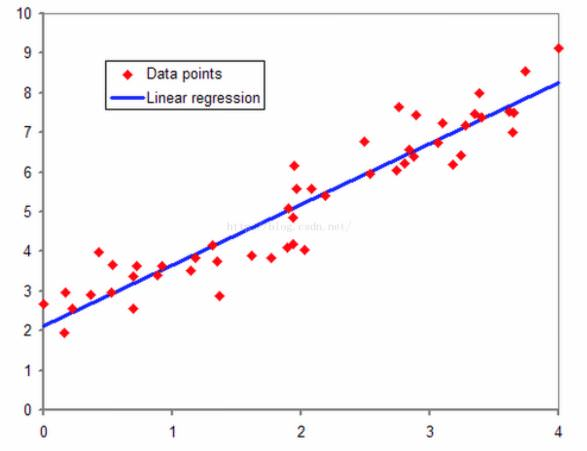2. 逻辑回归的由来

对于二类线性可分的数据集，使用线性感知器就可以很好的分类。如下图中红色和蓝色的点，我们使用一条直线

x

1

+

x

2

=

3

x_1 +x_2 = 3

就可以区分两种数据集，在直线上方的属于红色类，直线下方的属于蓝色类。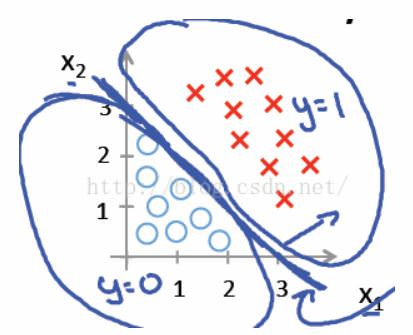但是如果二类线性不可分的数据集，我们无法找到一条直线能够将两种类别很好的区分，即线性回归的分类法对于线性不可分的数据无法有效分类。例如下图中的红色点和蓝色点，我们无法使用一条直线很好的区分这两类，但是我们可以使用非线性分类器，如果我们使用

x

1

2

+

x

2

2

=

1

{x_1}^2+{x_2}^2 = 1

，在圆外面的为红色类，在圆里面的一类为蓝色类。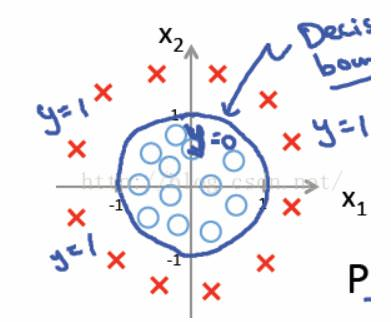诚然，数据线性可分可以使用线性分类器，如果数据线性不可分，可以使用非线性分类器，这里似乎没有逻辑回归什么事情。但是如果我们想知道对于一个二类分类问题，对于具体的一个样例，我们不仅想知道该类属于某一类，而且还想知道该类属于某一类的概率多大,有什么办法呢？  线性回归和非线性回归的分类问题都不能给予解答，因为线性回归和非线性回归的问题，假设其分类函数如下：

y

=

w

x

+

b

y = wx+b

y的阈值处于

（

−

∞

，

+

∞

）

（-\infty，+\infty）

，此时不能很好的给出属于某一类的概率，因为概率的范围是[0,1],我们需要一个更好的映射函数，能够将分类的结果很好的映射成为[0,1]之间的概率，并且这个函数能够具有很好的可微分性。在这种需求下，人们找到了这个映射函数，即逻辑斯谛函数，也就是我们常说的sigmoid函数，其形式如下：

1

1

+

e

−

z

\frac{1}{1+e^{-z}}

sigmoid函数图像如下图所示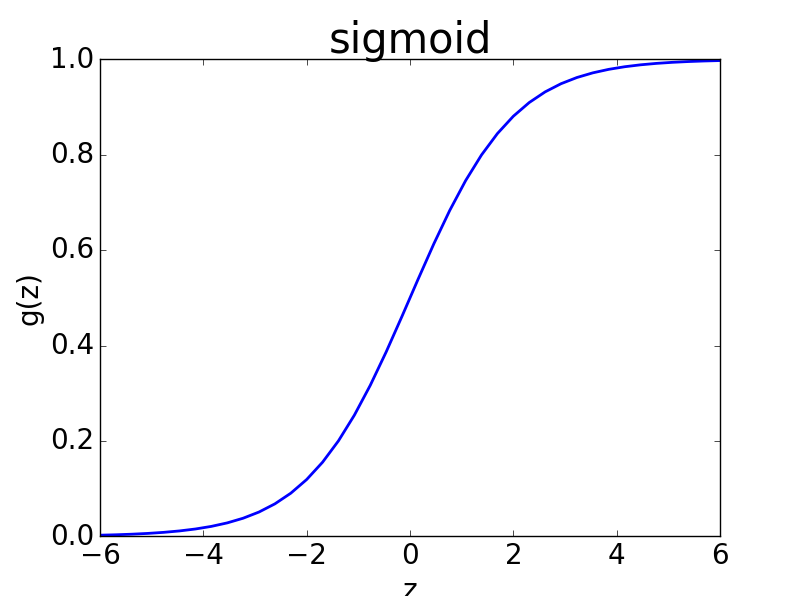sigmoid函数完美的解决了上述需求，而且sigmoid函数连续可微分。假设数据离散二类可分，分为0类和1类,如果概率值大于1/2，我们就将该类划分为1类，如果概率值低于1/2,我们就将该类划分为0类。当z取值为0的时候，概率值为1/2，这时候需要人为规定划分为哪一类。
3. 逻辑回归的损失函数(Loss Function)和成本函数(Cost Function)

在二类分类中，我们假定sigmoid输出结果表示属于1类的概率值，我们很容易想到用平方损失函数，即在这种情况下，我们φ(z(i))表示sigmoid对第i个值的预测结果，我们将sigmoid函数带入上述成本函数中，绘制其图像，发现这个成本函数的函数图像是一个非凸函数，如下图所示，这个函数里面有很多极小值，如果采用梯度下降法，则会导致陷入局部最优解中,有没有一个凸函数的成本函数呢？假设sigmoid函数φ(z)表示属于1类的概率，于是做出如下的定义：将两个式子综合来，可以改写为下式：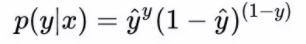上式将分类为0和分类和1的概率计算公式合二为一。假设分类器分类足够准确，此时对于一个样本，如果它是属于1类，分类器求出的属于1类的概率应该尽可能大，即p(y=1lx)尽可能接近1；如果它是0类，分类器求出的属于0类的概率应该尽可能大，即p(y=0lx)尽可能接近1。  通过上述公式对二类分类的情况分析，可知我们的目的是求取参数w和b，使得p(ylx)对0类和1类的分类结果尽可能取最大值，然而实际上我们定义的损失函数的是求最小值，于是，很自然的，我们想到对p(ylx)式子加一个负号，就变成了求最小值的问题，这就得到了逻辑回归中的损失函数。  不过，为了计算方便，我们通常对上述式子取log，因而得到下式：公式(1)是对概率公式取log，公式(2)是对公式(1)取相反数。上述公式的函数图像如下图所示。这是一个凸函数（斜率是非单调递减的函数即凸函数），因此可以用梯度下降法求其最小值。根据损失函数是单个样本的预测值和实际值的误差，而成本函数是全部样本的预测值和实际值之间的误差，于是对所有样本的损失值取平均数，得到我们的成本函数：损失函数是凸函数，m个损失函数的和仍然是凸函数，因而可以用梯度下降法求最小值。
4. 极大似然法求解逻辑回归
还可以用我们熟知的统计学知识——极大似然法估计逻辑回归中的参数w和b，上述得到的logp(y|w,x),假设目前有m组样本，分别为

(

x

1

,

y

1

)

,

(

x

2

,

y

2

)

.

.

.

(

x

m

,

y

m

)

(x_1,y_1),(x_2,y_2)...(x_m,y_m)

，其中xi表示第i个样本的特征，yi表示第i个样本的类别，yi = 0或者1，利用极大似然法的原则，假设所有训练样本独立同分布，则联合概率为所有样本概率的乘积，即：对上述公式两边取对数，得到下述公式，是不是对这个公式优点熟悉呢？这个公式就是我们的成本函数的和，对于这个公式和成本函数来说，取平均值和不取平均值没有影响。按照极大似然法求极值的方法，分别对w的每个参数求偏导数使其为0，得到对数似然方程组，求解该方程，便可以到的w的参数。只是如果参数很多，求解方程组就会很复杂，此时可以考虑梯度下降法来求解。
5. 总结 - 逻辑回归最大的优势在于它的输出结果不仅可以用于分类，还可以表征某个样本属于某类别的概率。
逻辑斯谛函数将原本输出结果从范围

（

−

∞

，

+

∞

）

（-\infty，+\infty）

映射到(0,1)，从而完成概率的估测。  逻辑回归得判定的阈值能够映射为平面的一条判定边界，随着特征的复杂化，判定边界可能是多种多样的样貌，但是它能够较好地把两类样本点分隔开，解决分类问题。  求解逻辑回归参数的传统方法是梯度下降，构造为凸函数的代价函数后，每次沿着偏导方向(下降速度最快方向)迈进一小部分，直至N次迭代后到达最低点。
参考： http://blog.csdn.net/han_xiaoyang/article/details/49123419 http://blog.csdn.net/zjuPeco/article/details/77165974 http://blog.csdn.net/star_liux/article/details/39666737
展开全文机器学习
• 1 逻辑等价理解 1.1 分配律 1.1.1 分配律1 假设你要找一个女孩子结婚，但是父母亲死活不同意，那么为了你能结婚，你必须说动你爸妈，或者你离家出走。 p：你离家出走 q：父亲同意 r：母亲同期 左侧：要么你离家...
离散数学
1 逻辑等价理解
1.1 分配律
1.1.1 分配律1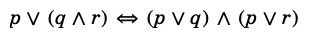假设你要找一个女孩子结婚，但是父母亲死活不同意，那么为了你能结婚，你必须说动你爸妈，或者你离家出走。
p：你离家出走q：父亲同意r：母亲同期
左侧：要么你离家出走，要么你爸妈都同意
右侧：你拿离家出走跟你爸沟通，你再拿离家出走跟你妈沟通

这里的情况就很简单了，为了追求真，我们要么离家出走，要么父母亲都同意。
1.1.2 分配律2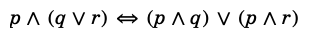p：成年q：父亲签字r：母亲签字
左侧：你成年，并且有父亲或者母亲的签字，才能参军
右侧：你成年，并且有父亲的签字。或者。你成年，并且有母亲的签字。这两项满足一项，你就可以去参军了。

这个是不是理解起来更加简单。
1.2 德摩根定律
1.2.1 德摩根定律1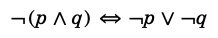可以配合这张图片来理解：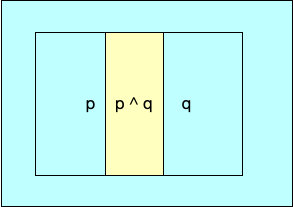1.2.2 德摩根定律2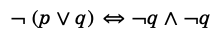可以配合这张图片理解：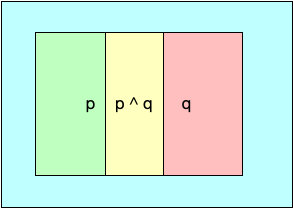1.3 吸收律
1.3.1 吸收律1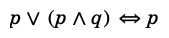1.3.2 吸收律2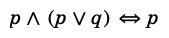上面两个定律都可以配合这张图片来理解：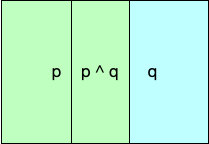展开全文• java逻辑习题先把12个球分成2组，每组6个，分别把这2个组重量相加然后比较2个组重量的大小java习题
• 在工作中有些平台需要对物理表进行逻辑处理，平台的功能基于逻辑表进行进一步拓展，逻辑表和物理表的概念...特殊的物理表不含有key逻辑表：逻辑表可以理解为数据库中的视图，是一张虚拟表。可以映射到一张物理表，...
在工作中有些平台需要对物理表进行逻辑处理，平台的功能基于逻辑表进行进一步拓展，逻辑表和物理表的概念如下：

物理表：物理表是具体某个数据源中的一张表。对于mysql就是一个table，对于Hbase可以是一张hbase表，对于ES是一个索引。mysql， Hbase和ES这些物理表必须要有合理的key。特殊的物理表不含有key
逻辑表：逻辑表可以理解为数据库中的视图，是一张虚拟表。可以映射到一张物理表，也可以由多张物理表组成，这些物理表可以来自于不同的数据源。对于mysql, Hbase和ES，要组成一张逻辑表，只需要他们有相同含义的key即可。这个key在mysql中是主键，Hbase中是生成rowkey用的值，是ES中的key。

展开全文• 逻辑回归（logisitc regression） 逻辑回归（logisitc regression） 1.逻辑分布 2.逻辑回归模型概念 3.极大似然估计 4.代价函数与损失函数 5.对数几率（log odds） 6.梯度下降 7.LR如何处理过拟合问题？ 8.多分类...
• 主要介绍了C语言的逻辑控制,对C语言的逻辑控制有较为深入的剖析，需要的朋友可以参考下
• 描述正逻辑和负逻辑，等效转换等，说明前后小圆圈有不同的含义，并指出具体如正负逻辑的变换条件，还有多级复合逻辑的变换等。
• 1.三个逻辑运算符应该怎样理解.好比逻辑与&&,它在与其它值作怎样的比较,大小,高低等? 2.逻辑运算在比较之后的取值,逻辑运算结果为假时,也就是为"0"时.关系不成立,不在计算.那结果为真时,也就为"1"时,关系成立,接着...
•java 习题
•java编程
• 一：逻辑删除 逻辑删除的本质是修改操作，所谓的逻辑删除其实并不是真正的删除，而是在表中将对应的是否删除标识（is_delete）或者说是状态字段（status）做修改操作。比如0是未删除，1是删除。在逻辑上数据是被删除...删除
• 北大青鸟 accp6.0的 s1课件----- 使用java理解程序逻辑
• 其中包括了很多非常有意思且经典的趣味逻辑题，有一些还出现在有些公司的面试题中，值得一看，可以锻炼一下思维，每一道题都有分析与解答。
• 这是一个完整的答案 包含从第一章到最后一章的课堂和课后的所有答案
• 1.回归和分类任务 分类和回归都属于监督学习（训练样本带有信息标记，利用已有的训练样本信息学习数据的规律预测未知的新样本标签） ...从名字来理解逻辑回归.在逻辑回归中,逻辑一词是logis...
• Java是一门面向对象编程语言，不仅吸收了C++语言的各种优点，还摒弃了C++里难以理解的多继承、指针等概念，因此Java语言具有功能强大和简单易用两个特征。Java语言作为静态面向对象编程语言的代表，极好地实现了面向...java 迷你DVD...